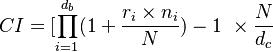# Difference between revisions of "Compound Interest Formula"

## Definition

Compound interest is the cumulation of interest to the principal sum of a loan or deposit

## Formula$CI = [ \prod_{i=1}^{d_b} (1 + \frac{r_i \times n_i}{N}) - 1 \ \times \frac{N}{d_c}$